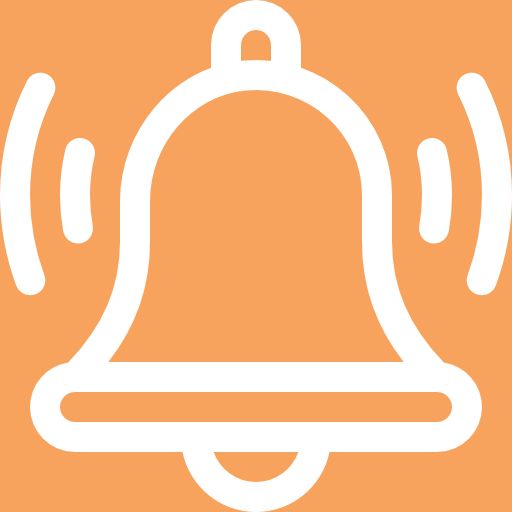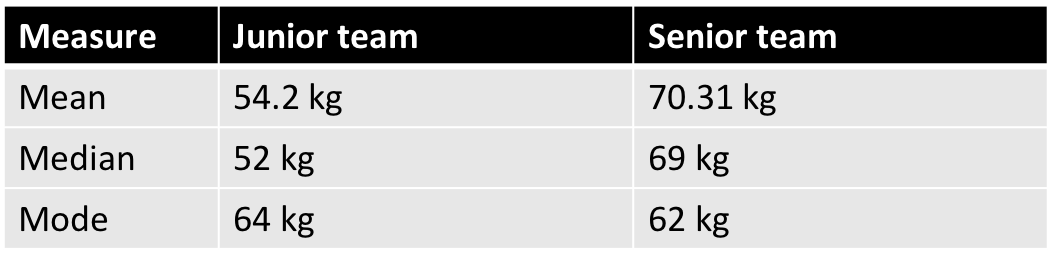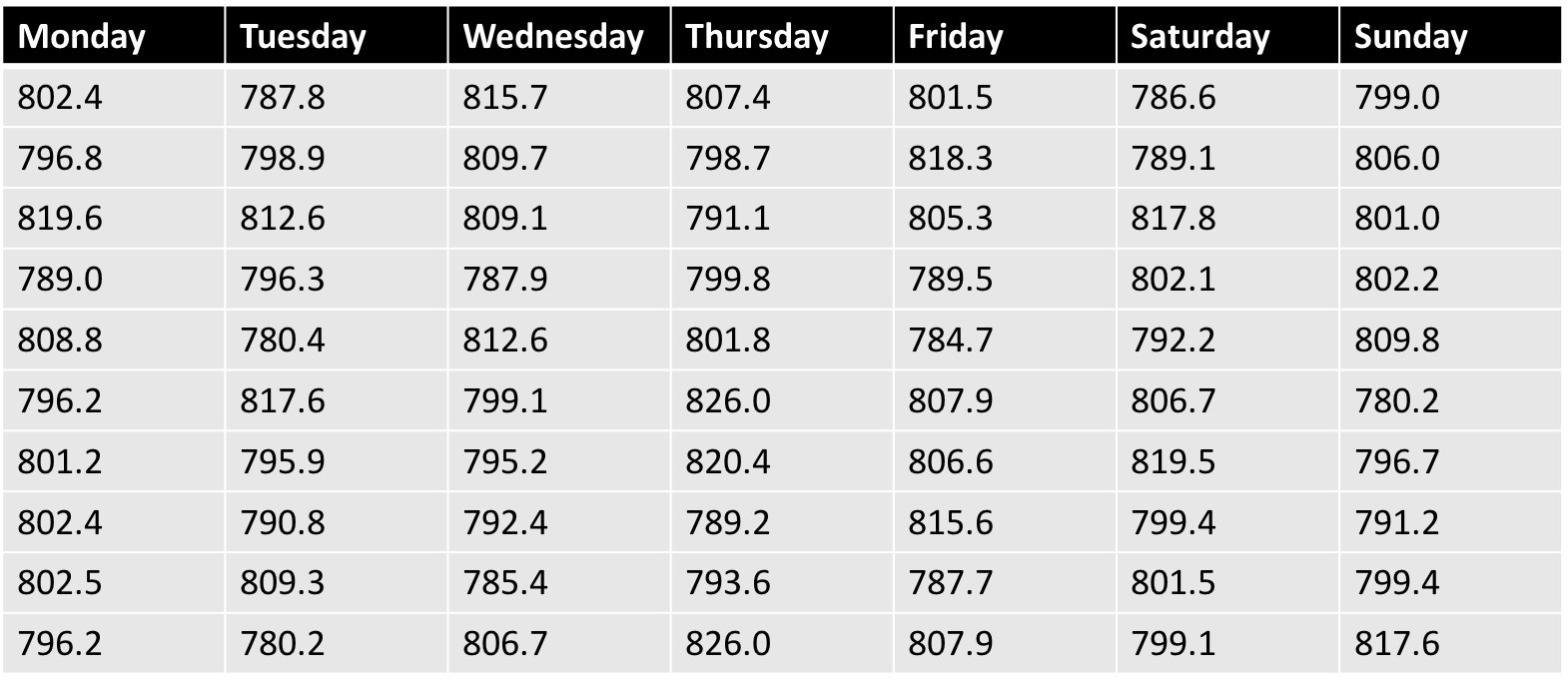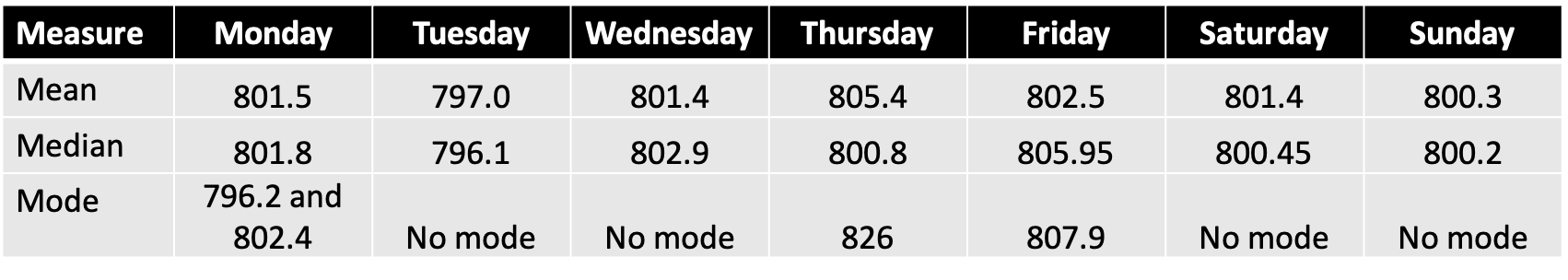Statistical and probability models: Calculate central tendencies and dispersion of data

# Unit 2: Measures of central tendency of ungrouped data

Dylan Busa### Unit 2: Measures of central tendency of ungrouped data

By the end of this unit you will be able to:

• Differentiate between the mean, median and mode.
• Calculate the mean, median and mode for ungrouped data.

## What you should know

Before you start this unit, make sure you can:

• Define the types of data.
• Differentiate between grouped and ungrouped data.

If you need help with this, review Unit 1 in this subject outcome.

## Introduction

Have a look at the test marks of learners in two different groups.

Group A:
$\scriptsize \{45\%,\text{ }60\%,\text{ }65\%,\text{ }72\%,\text{ }85\%,\text{ }67\%,\text{ }74\%,\text{ }87\%,\text{ }24\%,\text{ }36\%,\text{ }55\%\}$

Group B:
$\scriptsize \{48\%,\text{ }52\%,\text{ }68\%,\text{ }76\%,\text{ }83\%,\text{ }73\%,\text{ }69\%,\text{ }38\%,\text{ }75\%,\text{ }75\%,\text{ }79\%,\text{ }81\%\}$

On ‘average’ did Group A do better than Group B? It’s hard to tell by just looking at the raw data. We need a way to statistically compare them. Luckily, we have a number of statistical tools we can use to find where the middle or the average of a set of data lies. These are called measures of central tendency.

## Calculating measures of central tendency

Measures of central tendency are single numbers that provide summary information about an entire set of data, without listing every single data value. These single values represent the middle or centre values of the data and are helpful in comparing different sets of data. There are three main measures of central tendency: the mean, median and mode. We use different methods to calculate measures of central tendency for ungrouped and grouped data.

### The mean

You are probably familiar with the concept of an average. You may have calculated your average test or exam result to see how well you have done. You add all your results together and then divide by the total number of results.

What you are actually calculating is the mean of the marks. We can calculate the mean result for each group above as a way to compare them. Work out what the mean result of each of these groups is.

Group A:
\scriptsize \begin{align*}&\displaystyle \frac{{45\%+60\%+65\%+72\%+85\%+67\%+74\%+87\%+24\%+36\%+55\%}}{{11}}\\&=\displaystyle \frac{{670}}{{11}}\\&=60.9\%\end{align*}

Group B:
\scriptsize \begin{align*}&\displaystyle \frac{{48\%+52\%+68\%+76\%+83\%+73\%+69\%+38\%+75\%+75\%+79\%+81\%}}{{12}}\\&=\displaystyle \frac{{817}}{{12}}\\&=68.1\%\end{align*}

Group A had a mean mark of $\scriptsize 60.9\%$ and Group B had a mean mark of $\scriptsize 68.1\%$. Therefore, we can say that, on average, Group B did better.

Mean

The mean is the sum of a set of values, divided by the number of values in the set.

It can be expressed in mathematical notation as $\scriptsize \bar{x}=\displaystyle \frac{1}{n}\sum\limits_{{i=1}}^{n}{{{{x}_{i}}}}=\displaystyle \frac{{{{x}_{1}}+{{x}_{2}}+...+{{x}_{n}}}}{n}$
where $\scriptsize \bar{x}$ is the symbol used for mean and $\scriptsize \sum\limits_{{i=1}}^{n}{{{{x}_{i}}}}$ means add up all $\scriptsize x$ values in the set from the first ($\scriptsize i=1$) to the last ($\scriptsize i=n$).

### The median

Another measure of central tendency we can use to compare data sets is the median. The median is that value in the data set that splits the whole data set into a lower half and an upper half. To work out the median, we first need to sort the elements in the data set in ascending order.

So, to calculate the median of the Group A results above, we do the following:

1. List the results in ascending order.
$\scriptsize 24\%, 36\%, 45\%, 55\%, 60\%, 65\%, 67\%, 72\%, 74\%, 85\%, 87\%$
2. Find the middle value that splits the whole data set into a lower half and an upper half.
$\scriptsize 24\%, 36\%, 45\%, 55\%, 60\%, \boxed{65\%}, 67\%, 72\%, 74\%, 85\%, 87\%$

The median value of Group A is $\scriptsize 65\%$.

In this case, finding the median was simple because there were an odd number ($\scriptsize 11$) of values. But what happens when a data set has an even number of values, like Group B?

1. List the results in ascending order.
$\scriptsize 38\%, 48\%, 52\%, 68\%, 69\%, 73\%, 75\%, 75\%, 76\%, 79\%, 81\%, 83\%$
2. Find the middle two values that split the whole data set into a lower half and an upper half.
$\scriptsize 38\%, 48\%, 52\%, 68\%, 69\%, \boxed{73\%, 75\%}, 75\%, 76\%, 79\%, 81\%, 83\%$
3. Find the mean of these two values.
$\scriptsize \displaystyle \frac{{73+75}}{2}=\displaystyle \frac{{148}}{2}=74$

The median value of Group B is $\scriptsize 74\%$.### Take note!

Median

The median is the middle value, when the data set has been arranged from the lowest to the highest value.

### The mode

The mode is that value that is repeated most often in a data set. If there is no one value that is repeated most often, then a data set has no mode or is multi-modal (as more than one mode).

Do Group A and Group B have modes. If so, what are these?

In Group A, there is no one value that is repeated most often, In fact, all values appear only once. In Group B, however, $\scriptsize 75\%$ is repeated – it appears twice. Every other value appears only once. Therefore, Group B has a mode and it is $\scriptsize 75\%$.

The mode is not used very often when describing data, but it can be useful in certain circumstances. It is an appropriate measure to use with qualitative data, for example, a survey on car colour preferences.### Take note!

Mode

The mode is that value that occurs most often in the set. The mode is the most frequent or most common value in the data set.

### Note

Most often a continuous data set will have no mode. Since continuous values can lie anywhere on the real line, any particular value will almost never repeat. This means that the frequency of each value in the data set will be $\scriptsize 1$ and that there will be no mode.### Example 2.1

A high school has two cricket teams: a junior and a senior team. The junior team consists of $\scriptsize 17$ players (including reserves) and the senior team consists of $\scriptsize 16$ players (including reserves). The mass of each team member is given below. Use the data to answer the questions that follow.

Junior team masses (kg)
$\scriptsize \{56,\ 60,\ 67,\ 45,\ 51,\ 53,\ 64,\ 49,\ 56,\ 48,\ 42,\ 51,\ 64,\ 52,\ 64,\ 49,\ 50\}$

Senior team masses (kg)
$\scriptsize \{88,\ 81,\ 53,\ 62,\ 83,\ 68,\ 70,\ 62,\ 91,\ 78,\ 64,\ 74,\ 73,\ 54,\ 62,\ 62\}$

1. What is the mean mass of the senior team?
2. Arrange the masses of the senior team in ascending order.
3. Determine the mode of the senior team.
4. Determine the median of the senior team.
5. Calculate the mean of the masses of the junior team correct to one decimal digit.
6. Calculate the median of the masses of the junior team.
7. Calculate the mode of the masses of the junior team.
8. Look at the answers you found for the junior and senior teams. Which measure do you think gives the best measure of the real ‘average’ of each data set?

Solutions

1. .
\scriptsize \begin{align*}&\text{Mean}\\&=\displaystyle \frac{{88+81+53+62+83+68+70+62+91+78+64+74+73+54+62+62}}{{16}}\\&=\displaystyle \frac{{1\ 125}}{{16}}\\&=70.31\ \text{kg}\end{align*}
2. $\scriptsize 53,\ 54,\ 62,\ 62,\ 62,\ 62,\ 64,\ 68,\ 70,\ 73,\ 74,\ 78,\ 81,\ 83,\ 88,\ 91$
3. $\scriptsize 53, 54, \boxed{62, 62, 62, 62}, 64, 68, 70, 73, 74, 78, 81, 83, 88, 91$
$\scriptsize 62$ is the value that appears most often (four times) in the data set. Therefore, the mode is $\scriptsize 62\ \text{kg}$.
4. $\scriptsize 53, 54, 62, 62, 62, 62, 64, \boxed{68, 70}, 73, 74, 78, 81, 83, 88, 91$
$\scriptsize \displaystyle \frac{{68+70}}{2}=\displaystyle \frac{{138}}{2}=69$
Therefore, the median is $\scriptsize 69\ \text{kg}$.
5. .
\scriptsize \begin{align*}&\text{Mean}\\&=\displaystyle \frac{{56+60+67+45+51+53+64+49+56+48+42+51+64+52+64+49+50}}{{17}}\\&=\displaystyle \frac{{921}}{{17}}\\&=54.2\text{ kg}\end{align*}
6. $\scriptsize 42, 45, 48, 49, 49, 50, 51, 51, \boxed{52}, 53, 56, 56, 60, 64, 64, 64, 67$
The median is $\scriptsize 52\ \text{kg}$.
7. $\scriptsize 42, 45, 48, 49, 49, 50, 51, 51, 52, 53, 56, 56, 60, \boxed{64, 64, 64}, 67$
The mode is $\scriptsize 64\ \text{kg}$, because this value appears most often.
8. The following table contains a summary of the measures of central tendency for each team.We can see that, for both teams, the median value is close to the mean, but the modes are not. Of all these measures, therefore, either the mean or the median seems to be the best representations of the ‘average’ player mass.

The mode of the junior team is actually higher than the mode of the senior team and is much greater than either the mean or the median. The mode of the senior team is much less than either the mean or the median.

In Example 2.1, we looked at the masses of players in a sports team. Generally, due to natural variations we would expect all the players to have different weights. However, we would also expect these measures to be more or less equally distributed about the mean, with half of them being less than the mean and half of them being greater than the mean. We call this a normal distribution. This is illustrated in Figure 1.

In a normal distribution, because all the values of the data set are equally distributed above and below the mean, the median tends to be the same (or similar to) the mean. The data is said to have no skew. If there is a mode, it is also most often similar to the mean and the median as well (but not necessarily so).

However, in some cases, many more of the data values actually lie above the mean with a few extreme values below the mean. In this case, the median value is greater than the mean. Because the ‘long tail’ of the few, but extreme, values are on the ‘negative’ side of the mean, we call this a negative skew. Any value that is very different from the majority of the other values in a data set is called an outlier.### Take note!

Outlier

An outlier is a value in the data set that is not typical of the rest of the set. It is usually a value that is much greater or much smaller than most of the other values in the data set.

The opposite can also happen. A positively skewed data set has a long tail of a few relatively extreme values on the positive side of the mean. In this case the median is less than the mean.

If the mean and median of a data set are very different, this could indicate that the data set is skewed in some way.

If you have an internet connection, watch this excellent video on the subject of skewness called “Statistics: Skewness and Measures of Center”.

Statistics: Skewness and Measures of Center (Duration: 04.14)### Take note!

Skewness

If the value of the calculation of the difference between mean and median (mean – median) is very close to 0, the data set is symmetrical.

If the value of the calculation of mean − median is greater than 0 (or positive), the data is skewed right or is positively skewed.

If the value of the calculation of mean − median is less than 0 (or negative), the data is skewed left or is negatively skewed.### Exercise 2.1

1. South African regulations stipulate that, if the mass of a loaf of bread is not given, it must weigh between $\scriptsize 760\ \text{g}$ and $\scriptsize 880\ \text{g}$. The mass of ten newly baked loaves of bread were recorded each day for one week. The results, in grams, are given in the following table.2. Determine the mean, median and mode of the mass of the loaves of bread for each day of the week. Give your answers correct to one decimal place and summarise your findings in a table.
3. Based on the data, do you think that this supplier is providing bread within the South African regulations? Justify your answer.
2. The heights of $\scriptsize 11$ girls in a netball team are measured in centimetres. The data set is as follows:
$\scriptsize \{151,\ 171,\ 153,\ \text{147},\ 142,\ 167,\ 146,\ 157,\ 156\text{, }157,\ 158\}$
1. Calculate the mean, median and mode of the data.
2. Is the data skewed? If so, how?
3. A twelfth player is added to the squad who is exceptionally tall at $\scriptsize 183\ \text{cm}$. Recalculate the mean, median and mode for the new dataset and explain any changes (or not) to these measures.
4. Is the new data set skewed? If so, how?

The full solutions are at the end of the unit.### Example 2.2

A group of $\scriptsize 13$ friends each have some marbles. They work out that the mean number of marbles they have is $\scriptsize 12$. Then seven friends leave with an unknown number ($\scriptsize x$) of marbles. The remaining six friends work out that the mean number of marbles they have left is $\scriptsize 15.5$. How many marbles did the seven friends take with them?

Solution

We can calculate the total number of marbles the group of $\scriptsize 13$ had.

\scriptsize \begin{align*}\bar{x}=\displaystyle \frac{{{{x}_{1}}+{{x}_{2}}+...+{{x}_{{13}}}}}{{13}} & =12\\\therefore {{x}_{1}}+{{x}_{2}}+...+{{x}_{{13}}} & =12\times 13\\ & =156\end{align*}

After the seven leave, the mean number of marbles is $\scriptsize 15.5$. Therefore, we can calculate the new total number of marbles.

\scriptsize \begin{align*}\bar{x}=\displaystyle \frac{{{{x}_{1}}+{{x}_{2}}+...+{{x}_{6}}}}{6} & =15.5\\\therefore {{x}_{1}}+{{x}_{2}}+...+{{x}_{6}} & =15.5\times 6\\ & =93\end{align*}

Therefore, the seven friends took $\scriptsize 156-93=63$ marbles with them.### Exercise 2.2

1.  A group of $\scriptsize 27$ employees have a mean monthly income of $\scriptsize \text{R}17\ 510$. Three employees resign and the mean monthly income drops to $\scriptsize \text{R}16\ 113$. What was the mean monthly income of the three employees who resigned?
2. While doing a fuel economy test, a driver makes $\scriptsize 16$ test drives. The mean amount of fuel consumed during each test drive was $\scriptsize 7.72\ \ell$. If the four least economical journeys are removed from the data set, the mean fuel consumption drops to $\scriptsize 6.96\ \ell$ per test drive.
1. What was the mean fuel consumption of these four test drives?
2. If three of the $\scriptsize 12$ most efficient test drives each consumed $\scriptsize 8\ \ell$ of fuel, would you say the data is skewed and, if so, how?
3. Find a set of eight ages less than or equal to $\scriptsize 10$ for which the mean age is $\scriptsize 4.75$, the modal age is two and the median age is four years.

The full solutions are at the end of the unit.

## Summary

In this unit you have learnt the following:

• There are three simple ways to represent the ‘middle’ of a quantitative data set – the mean, the median and the mode.
• The mean is the sum of all the values divided by the number of values.
• The median is the middle value when the values are arranged from smallest to biggest.
• The mode is the value that appears most often (with the highest frequency).
• If the value of the calculation of mean − median is very close to 0, the data set is symmetrical.
• If the value of the calculation of mean − median is greater than 0 (or positive), the data is skewed right or is positively skewed.
• If the value of the calculation of mean − median is less than 0 (or negative), the data is skewed left or is negatively skewed.

# Unit 2: Assessment

#### Suggested time to complete: 30 minutes

1. The ages of 20 cyclists in the Cape Argus Cycle race were recorded. Calculate the mean, median and modal age.
$\scriptsize \{31,\ 42,\ 28,\ 38,\ 67,\ 43,\ 45,\ 51,\ 33,\ 5\text{3},\ 29,\ 42,\ 26,\ 34,\ 35,\ 56,\ 33,\ 43,\ 46,\ 41\}$
2. A group of $\scriptsize 15$ salespeople were surveyed on the total value of their sales of a product in the previous month. The data is given below. Answer the following questions based on this data.
$\scriptsize \text{R}13\ 346,\ \text{R}14\ 341,\ \text{R}14\ 416,\ \text{R24}\ 512,\ \text{R36}\ 973,\ \text{R}12\ 014,\ \text{R4}3\ 852,\ \text{R40}\ 915,\\\text{R16}\ 536,\ \text{R82}\ 366,\ \text{R17}\ 340,\ \text{R28}\ 361,\ \text{R}130\ 011,\ \text{R}14\ 815,\ \text{R24}\ 836$
1. What is the mean, median and mode of this data?
3. If it came to light that the highest value was actually misreported and should have been reported as $\scriptsize \text{R}13\ 011$ instead, would you still consider the data to be skewed? Explain your answer.
3. Four friends each have some marbles. They work out that the mean number of marbles they have is $\scriptsize 10$. One friend leaves with four marbles. What is the new mean number of marbles of the three remaining friends?

The full solutions are at the end of the unit.

# Unit 2: Solutions

### Exercise 2.1

1. .
1. The data is quantitative. It consists of numerical values of the mass of loaves of bread.
2. .3. The regulations state that each loaf must be between $\scriptsize 760\ \text{g}$ and $\scriptsize 880\ \text{g}$. On each day, the mean and median were very close to each other and to $\scriptsize 800$ indicating that the data is not significantly skewed negative or positive. Where modes are present, these are close to, or greater than, $\scriptsize 800$. The summary data does not specifically indicate or identify any outliers, but the summary data does seem to suggest that the manufacturer is well within the regulations.
.
Looking at the raw data, one can see that all values do fall within the stipulated range.
2. $\scriptsize \{151,\ 161,\ 153,\ \text{147},\ 142,\ 167,\ 146,\ 157,\ 156\text{, }157,\ 158\}$
1. Mean: $\scriptsize 155\ \text{cm}$
Median: $\scriptsize 142, 146, 147, 151, 153, \boxed{156}, 157, 157, 158, 167, 171$. The median is $\scriptsize 156\ \text{cm}$.
Mode: 3. $\scriptsize 142, 146, 147, 151, 153, 156, \boxed{157, 157}, 158, 167, 171$. The mode is $\scriptsize 157\ \text{cm}$.
2. The mean and median are fairly close to each other. That the median is less than the mean indicates that the data is positively skewed. The fact that the mode is $\scriptsize 157$ indicates that there are some players significantly below the mean height.
3. Mean: $\scriptsize 157.3\ \text{cm}$
Median: $\scriptsize 142, 146, 147, 151, 153, \boxed{156, 157}, 157, 158, 167, 171$. Therefore, the median is $\scriptsize 156.5\ \text{cm}$.
Mode: $\scriptsize 142, 146, 147, 151, 153, 156, \boxed{157, 157}, 158, 167, 171$. The mode is $\scriptsize 157\ \text{cm}$.
4. In this case, even with this new outlier, the mean and median are much closer together, indicating less overall skew than previously. The mean, median and mode are also all much more similar. Hence even though there is now a clear outlier, this value seems to balance out the heights that are significantly below the mean.

Back to Exercise 2.1

### Exercise 2.2

1. Total earnings before employees left:
\scriptsize \begin{align*}\bar{x}=\displaystyle \frac{{{{x}_{1}}+{{x}_{2}}+...+{{x}_{{27}}}}}{{27}} & =17\ 510\\\therefore {{x}_{1}}+{{x}_{2}}+...+{{x}_{{27}}} & =17\ 510\times 27\\ & =472\ 770\end{align*}
Total earnings after employees left:
\scriptsize \begin{align*}\bar{x}=\displaystyle \frac{{{{x}_{1}}+{{x}_{2}}+...+{{x}_{{24}}}}}{{24}} & =16\ 113\\\therefore {{x}_{1}}+{{x}_{2}}+...+{{x}_{{24}}} & =16\ 113\times 24\\ & =386\ 712\end{align*}
Total earnings of three employees:
$\scriptsize 472\ 770-386\ 712=86\ 058$
Mean earnings of three employees:
$\scriptsize \bar{x}=\displaystyle \frac{{86\ 058}}{3}=28\ 686$
Therefore, the three employees who left earned a mean monthly salary of $\scriptsize \text{R}28\ 686$.
2. .
1. Total fuel consumption of all test drives:
\scriptsize \begin{align*}\bar{x}=\displaystyle \frac{{{{x}_{1}}+{{x}_{2}}+...+{{x}_{{16}}}}}{{16}} & =7.72\\\therefore {{x}_{1}}+{{x}_{2}}+...+{{x}_{{16}}} & =7.72\times 16\\ & =123.52\end{align*}
Total fuel consumption excluding four least efficient test drives:
\scriptsize \begin{align*}\bar{x}=\displaystyle \frac{{{{x}_{1}}+{{x}_{2}}+...+{{x}_{{12}}}}}{{12}} & =6.96\\\therefore {{x}_{1}}+{{x}_{2}}+...+{{x}_{{12}}} & =6.96\times 12\\ & =83.52\end{align*}
Total fuel consumption of four least efficient test drives:
$\scriptsize 123.52-83.52=40$
Mean fuel consumption of the four least efficient test drives:
$\scriptsize \bar{x}=\displaystyle \frac{{40}}{4}=10$
Therefore, the four least efficient test drives consumed an average of $\scriptsize 10\ \ell$ of fuel.
2. Yes, the data is skewed. It is negatively skewed. The mean of the four least efficient test drives is quite a bit greater than the overall mean of the data and three of the remaining $\scriptsize 12$ most efficient drives are higher than the mean of these $\scriptsize 12$ test drives as well.
3. A set of eight ages less than or equal to $\scriptsize 10$ for which the mean age is $\scriptsize 4.75$ , the modal age is 2 and the median age is 4 years.There are eight values in the set: $\scriptsize \boxed{}, \boxed{}, \boxed{}, \boxed{}, \boxed{}, \boxed{}, \boxed{}, \boxed{}$

The median is four: $\scriptsize \boxed{}, \boxed{}, \boxed{}, \boxed{3}, \boxed{5}, \boxed{}, \boxed{}, \boxed{}$
The mode is two: $\scriptsize \boxed{}, \boxed{2}, \boxed{2}, \boxed{3}, \boxed{5}, \boxed{}, \boxed{}, \boxed{}$

Therefore, the first value must be one: $\scriptsize \boxed{1}, \boxed{2}, \boxed{2}, \boxed{3}, \boxed{5}, \boxed{}, \boxed{}, \boxed{}$

The mean is $\scriptsize 4.75$. Therefore, the total ages must be $\scriptsize 4.75\times 8=38$.

Therefore, the total of the last three ages must be $\scriptsize 38-1-2-2-3-5=25$ and none of them can be the same (the mode is two).

Therefore, the data set must be $\scriptsize \boxed{1}, \boxed{2}, \boxed{2}, \boxed{3}, \boxed{5}, \boxed{6}, \boxed{9}, \boxed{10}$ or $\scriptsize \boxed{1}, \boxed{2}, \boxed{2}, \boxed{3}, \boxed{5}, \boxed{7}, \boxed{8}, \boxed{10}$

Back to Exercise 2.2

### Unit 2: Assessment

1. Mean: \scriptsize \begin{align*}\bar{x}&=\displaystyle \frac{{31+42+28+38+67+43+45+51+33+5\text{3+}29+42+26+34+35+56+33+43+46+41}}{{20}}\\&=40.8\end{align*}Median: $\scriptsize 26, 28, 29, 31, 33, 34, 35, 38, \boxed{41, 42}, 42, 43, 43, 45, 46, 51, 53, 56, 67$. Therefore, the median is $\scriptsize 41.5$.

Mode: $\scriptsize 33,42$ and $\scriptsize 43$ are each repeated twice. Therefore, there are three modal ages (the data is ‘trimodal’).

1. Mean: $\scriptsize \bar{x}=\displaystyle \frac{{\text{R}514\ 634}}{{15}}=\text{R}34\ 308.93$
Median:.
\scriptsize \begin{align} & \text{R}12\ 014,\ \text{R}13\ 346,\ \text{R}14\ 341,\ \text{R}14\ 416,\ \text{R}14\ 815,\ \text{R}16\ 536,\ \text{R}17\ 340, \boxed{\text{R}24\ 512},\\ & \text{R}24\ 836,\ \text{R}28\ 361,\ \text{R36}36\ 973,\ \text{R}40\ 915,\ \text{R}43\ 852,\ \text{R}82\ 366,\ \text{R}130\ 011 \\ \end{align}
The median value is $\scriptsize \text{R}24\ 512$.Mode: There is no mode
2. Yes, the data is skewed. It is positively skewed because the median is substantially less than the mean. Therefore, there are a few, but extreme, values to the right of the mean.
3. Mean: $\scriptsize \bar{x}=\displaystyle \frac{{\text{R397}\ 634}}{{15}}=\text{R26}\ 508.93$
Median:.
\scriptsize \begin{align} & \text{R}12\ 014,\ \text{R}13\ 011,\ \text{R}13\ 346,\ \text{R}14\ 341,\ \text{R}14\ 416,\ \text{R}14\ 815,\ \text{R16}\ 536, \boxed{\text{R}17\ 340},\\ & \text{R}24\ 512,\ \text{R}24\ 836,\ \text{R}28\ 361,\ \text{R}36\ 973,\ \text{R}40\ 915,\ \text{R}43\ 852,\ \text{R}82\ 366 \\ \end{align}
The median value is $\scriptsize \text{R}17\ 340$.With the correction of the data value, the mean value is reduced by about $\scriptsize \text{R}8\ 000$ to $\scriptsize \text{R26}\ 508.93$. This is similar to the original median value. However, the new median value is $\scriptsize \text{R}17\ 340$. Therefore, the median is still less than the mean although by a slightly smaller amount. Therefore, the data is still positively skewed but, perhaps, not as skewed.
2. Four friends:
\scriptsize \begin{align*}\bar{x}=\displaystyle \frac{{{{x}_{1}}+{{x}_{2}}+...+{{x}_{4}}}}{4} & =10\\\therefore {{x}_{1}}+{{x}_{2}}+...+{{x}_{4}} & =10\times 4=40\end{align*}
Three remaining friends will have $\scriptsize 40-4=36$ marbles.Therefore, the new mean is:
$\scriptsize \bar{x}=\displaystyle \frac{{36}}{3}=12$ marbles

Back to Unit 2: Assessment